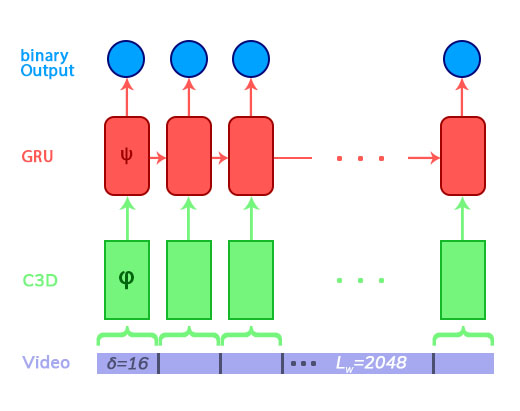# [RNN] Architecture Problems

Hey there, I am having some problems with a Video Detection RNN. My Architecture looks like the following:For training, I am having a video with `vid_length=2048` frames, I divide the the video into `vid_length / delta` separate blocks. These blocks are used as input for a `(3D-ConvNet) C3D-feature-extractor` so this `Conv3d net` is encoding the features from `delta=16` frames. I then `flatten` the output and use it as input for `GRU layer` this outputs a value between 0 - 1 which is the classification for the the `delta=16` frames at `time_step=n` (where `n` is from `1 to vid_lenght / delta`.

Now to my question: My `seq_length=512` frames ergo `feature_seq_length=32` so the input for the GRU is of size `(batch, 32, C3D_output_size)`. How do I process the input for the C3D so I that I have the 32 outputs as inputs for the GRU. I would like that the model is trainable end-to-end? My approach would be to run the C3D on the 512 frames to get 32 outputs and use those as a sequence for the GRU. What is your opinion on this?

I feel bad with tagging you here, but you are a nice person, right? Thanks for your previous help@ptrblck. Looking forward to contribute here, it’s an amazing forum!

We have a lot of awesome guys here, but thanks for the kind words.I don’t quite understand your second paragraph. Do you want to use only `512` frames for the GRU and then reset it?

I know. Hopefully I will be one of them soon too :D. So, during training time I have example videos of `vid_length=2048` frames but the max length my RNN rolls out is `seq_length=512` frames, because it takes the output from the `Conv3D` which itself acts as a feature-detector on `delta=16` frames so the actual input for the RNN is `seq_length=32` (32 * 16 = 512) because I have this `Conv3D` which ‘summarizes’ 16 frames into one feature map. a timestep `t_n` to `t_n+1` is 16 frames.

So I want to use 32 feature maps (512 frames) for the RNN and then reset it, yes.

OK, thanks for the info. I had a bit of spare time and tried to create a starter code:

``````class MyModel(nn.Module):
def __init__(self, window=16):
super(MyModel, self).__init__()
self.conv_model = nn.Sequential(
nn.Conv3d(
in_channels=3,
out_channels=6,
kernel_size=3,
stride=1,
nn.MaxPool3d((1, 2, 2)),
nn.ReLU()
)

self.rnn = nn.RNN(
input_size=6*16*12*12,
hidden_size=1,
num_layers=1,
batch_first=True
)

self.hidden = torch.zeros(1, 1, 1)

self.window = window

def forward(self, x):
self.hidden = torch.zeros(1, 1, 1) # reset hidden

activations = []
for idx in range(0, x.size(2), self.window):
x_ = x[:, :, idx:idx+self.window]
x_ = self.conv_model(x_)
x_ = x_.view(x_.size(0), 1, -1)
activations.append(x_)
x = torch.cat(activations, 1)
out, hidden = self.rnn(x, self.hidden)

return out, hidden

class MyDataset(Dataset):
def __init__(self, frames=512):
self.data = torch.randn(3, 2048, 24, 24)
self.frames = frames

def __getitem__(self, index):
index = index * self.frames
x = self.data[:, index:index+self.frames]
return x

def __len__(self):
return self.data.size(1) / self.frames

model = MyModel()
dataset = MyDataset()
x = dataset
output, hidden = model(x.unsqueeze(0))
``````

As I’m not that experienced with RNNs, you should definitely check for logical errors.
Let me know, if you find something weird.

Also, currently I’m using the output of the RNN. If you want, you could add a linear layer after the RNN, use a bigger hidden size, and return the linear layer with a sigmoid as the non-linearity. This would probably better fit your use case.

2 Likes

Wow thanks a lot, that is almost like I have implemented it, the dataset part is really helpful, WOW! <3<3<3 Gonna implement it further and will let you know!

1 Like